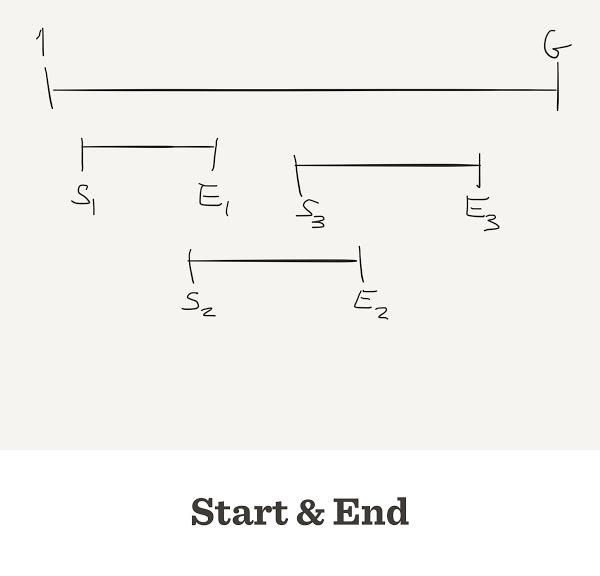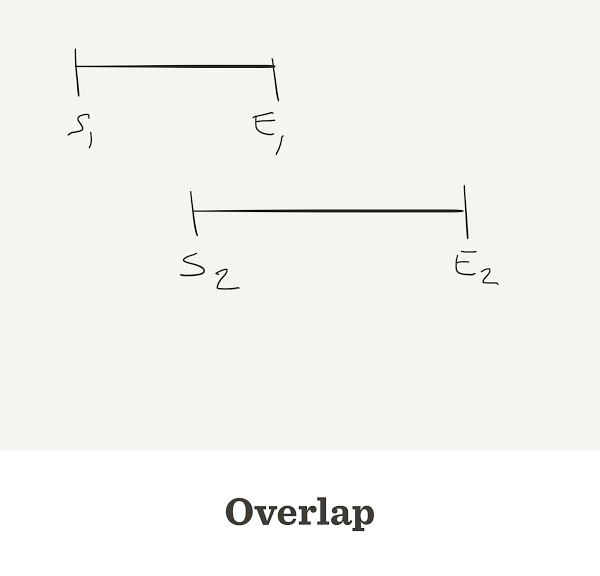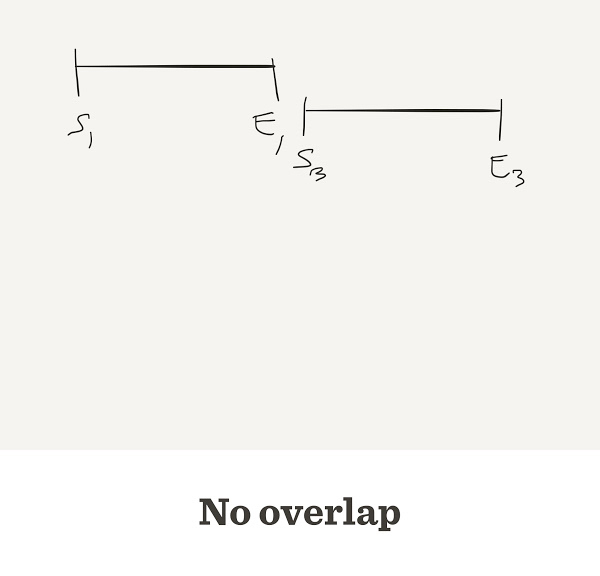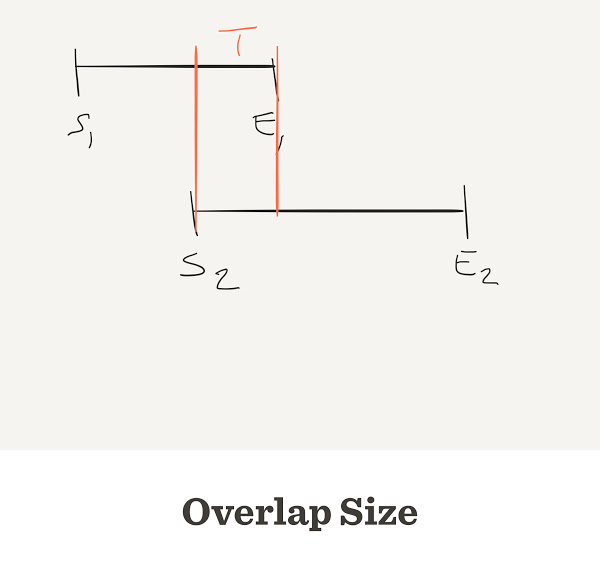October 2nd, 2018

## Assembly Statistics

The number of contigs depends on

• Genome length: G
• Length of reads: L
• Number of reads: N

In general L can be different for each read. In this simulation we will assume all reads have the same length

Exercise: Find the real values of L in our reads

## Each read has start and end## One function to simulate all

sim_sequencing <- function(N, L, G) {
start <- sample.int(G, size=N)
end <- start + L
depth <- rep(0, G)
for(i in 1:N) {
}
return(depth)
}

## Depth and Coverage

Depth is a property of each position in the genome

Depth average is also known as coverage

Coverage can be calculated before sequencing

get_coverage <- function(N, L, G) {
return(N*L/G)
}

This is the percentage of the genome that we can see with our reads

We can only know coverage breadth after we assembly

get_breadth <- function(depth, G, MIN=0) {
return(sum(depth>MIN)/G)
}

## The result changes every time## Simulate to get a confidence interval

The question we want to answer is

How many reads we need to see all the genome with a minimum depth?

How would you answer that question?

## How assemblers work

So far we have assumed that we know the exact position of the read in the geome

That is never true

What classic assemblers do is to find when the end of one read is equal to the start of another read

That is called overlap## Sometimes they do not overlap## What is the overlap size?## Distribution of overlap length

overlap <- rep(NA, N-1)
for(i in 1:(N-1)) {
overlap[i] <- end[i] - start[i+1]
}

## Let’s pack everything on one function

overlap_sizes <- function(N, L, G) {
start <- sample.int(G, N) # shotgun
start <- sort(start) # This is important
end <- start + L
overlap <- rep(NA, N-1)
for(i in 1:(N-1)) {
overlap[i] <- end[i] - start[i+1]
}
return(overlap)
}

Exercise: can we avoid the for() loop?

## Shorter version

overlap_sizes <- function(N, L, G) {
start <- sample.int(G, N) # shotgun
start <- sort(start) # This is important
end <- start + L
overlap <- end[-N] - start[-1]
return(overlap)
}

## Negative overlap are gaps## Two reads connect when they overlap

We only see overlaps over a threshold thr

The best thr has to be big enough to guarantee that the reads do not overlap by chance

Bigger values of thr reduce the probability of “overlap by chance”

## If two reads overlap, they are in the same Contig

A group of contiguous sequences is called Contig

The goal of the assembly process is to find one contig.

The sequence of this contig will be the genome

Most of times we do get several contigs

## Number of contigs depends on G, L, N and thr

num_contigs <- function(N, L, G, thr) {
num_gaps <- sum(overlap_sizes(N, L, G) < thr)
return(num_gaps)
}

## How many reads shall you pay?

num.reads <- seq(from=10, to=5E4, by=2E3)
G=1e6, thr=20)
}

## Result

plot(n.contigs ~ num.reads, type="b")## Faster version

num.reads <- seq(from=10, to=5E4, by=2E3)
G=1e6, thr=20)

## Result is the same

plot(n.contigs ~ num.reads, type="b")## What if reads are longer?

n.contigs <- sapply(num.reads, num_contigs, L=600, G=1e6, thr=20)
plot(n.contigs ~ num.reads, type="b")## Contig length

With this simulation we can also calculate the length of each contig.

contig_len
  149 1377  401 1363 1450  265 2001  351
sum(contig_len)
 7357

## N50

Given a set of contigs, the N50 is defined as the sequence length of the shortest contig at 50% of the total genome length

We sort the contigs from largest to smallest

sort(contig_len, decreasing = TRUE)
 2001 1450 1377 1363  401  351  265  149

## Which contig reach 50% of bases?

sorted_len pct_in_contig cumulative
2001 27.20 27.20
1450 19.71 46.91
1377 18.72 65.62
1363 18.53 84.15
401 5.45 89.60
351 4.77 94.37
265 3.60 97.97
149 2.03 100.00